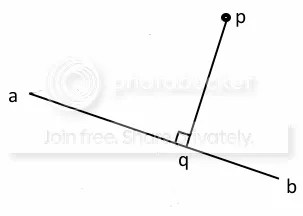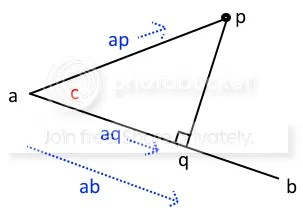I hate not understanding things, even if they require me to understand math. So I found a great explanation of how to calculate the shortest distance from a point to a line.

I got this explanation from a marvellous book, the Nature of Code, free to read online, which explains everything you need to move around in 2D. It uses the Processing language, which is so similar to Codea that you will have no trouble adapting it. If you haven’t read it, give it a try, as I think it’s the most clearly explained programming book I’ve read. Which is why I went there first when I was puzzling about this… The explanation I am adapting is from section “6.8 Path Following”.

So here goes. We have a line from a to b, and a point p, as shown below.To calculate the shortest point, we need to drop a line from p onto the line ab, at a right angle (which will give us the shortest distance). The point where the two lines meet is marked q. So we want the distance from p to q.

We are going to do it by first getting the distance between a and q, and then we know the position of q, and can calculate the distance from p to q.

In the diagram below, I have shown three blue markers.

• ap = p – a, ie the difference between a and p
• aq = q – a
• ab = b – a

So if a=vec2(3,4) and p=vec3(7,9), then p-a=vec2(4,5).

I’ve also shown in red, the angle c, between ap and aq.The length of the line from a to p is the length of the vector ap, written as |ap|.

Similarly, the length of the line from a to q is written as |aq|. And this is what we are going to try to find.

Trigonometry tells us that cos(c) = |aq| / |ap|, which means |aq| = |ap| cos(c), which would make things easy if we knew what the angle c was. But we don’t.

This is where the dot product comes in, because

ap DOT ab = |ab| |ap| cos(c)  (proof here)

and since |aq| = |ap| cos(c), then ap DOT ab = |ab| |aq|

and so |aq| = (ap DOT ab) / |ab|

We can simplify this a little by normalising ab before we do the dot product, because normalising divides ab by its length |ab|, and if we call the result AB, then |AB|=1, and so |aq| = (ap DOT AB). We’ll also need AB for something else, which is another good reason for calculating it.

Now we know the length of aq, we need to move that distance along the line ab to get to q, then we can calculate the distance to p.

q = a + AB * |aq|

This is because AB is the direction of the line from a to b, with length 1 (remember, we normalised it). So if we want to travel 5 pixels along the line, we multiply AB by 5. In our case, we multiply by |aq|, the length of the line from a to q.

So q = a + AB * (ap DOT AB), and then it is easy to calculate the distance to p.

There is just one more test. If the result is beyond a or b, then we restrict it to a or b.

Time for some code.

```function PointToLine(p,a,b)
local AB=(b-a):normalize()
local ap=(p-a)
local m=a:dist(b) --needed to check we don't go beyond the line
local s=math.max(0,math.min(m,ap:dot(AB)))
local q=a+s*AB
return p:dist(q),q --return distance and intersection point
end
```

How does this compare with the previous forum code, which looks like this?

```function lineDistB(p,a,b)
return (p-a):dist(math.max(math.min((p-a):dot(b-a)/
(b-a):lenSqr(),1),0)*(b-a))
end
```

Using my terminology above to turn this back into a formula, and ignoring the max/min checks, we have

distance = distance from ap to (ap DOT ab) / (|ab| |ab|) * ab

= distance from ap to (ap DOT AB) * (ab /|ab|)

= distance from ap to (ap DOT AB) * AB

my formula above is

= distance from p to q = distance from p to a + (ap DOT AB) * AB

subtracting a from both sides

= distance from p-a to (ap DOT AB) * AB

and since p-a = ap, it gives the same answer as the forum equation.

I did still write a test program to make sure it gave the answers to thousands of randomised examples, which it did. My program above was slightly faster than the one line forum version, seemingly because that version calculates b-a and p-a multiple times. Corrected for this, it is faster than mine, as I would expect.

So here is my amended version of the forum function, which returns both the distance, and q, the point where the shortest line from p meets the line a to b.

```--p is vec 2 point, a and b are the ends of the line, also vec2
--function returns distance from p to line, and the meeting point
function DistanceToLine(p,a,b)
local ap,ab=p-a,b-a
q= math.max(math.min(ap:dot(ab)/ab:lenSqr(),1),0)*ab+a
return p:dist(q),q
end
```

However, I’m splitting hairs, because my iPad 3 can do this calculation 1,000,000 times in about 30 seconds, so the speed difference is immaterial. But I think it’s a good idea to also pass back the point where p joins the line, because this can be useful sometimes.